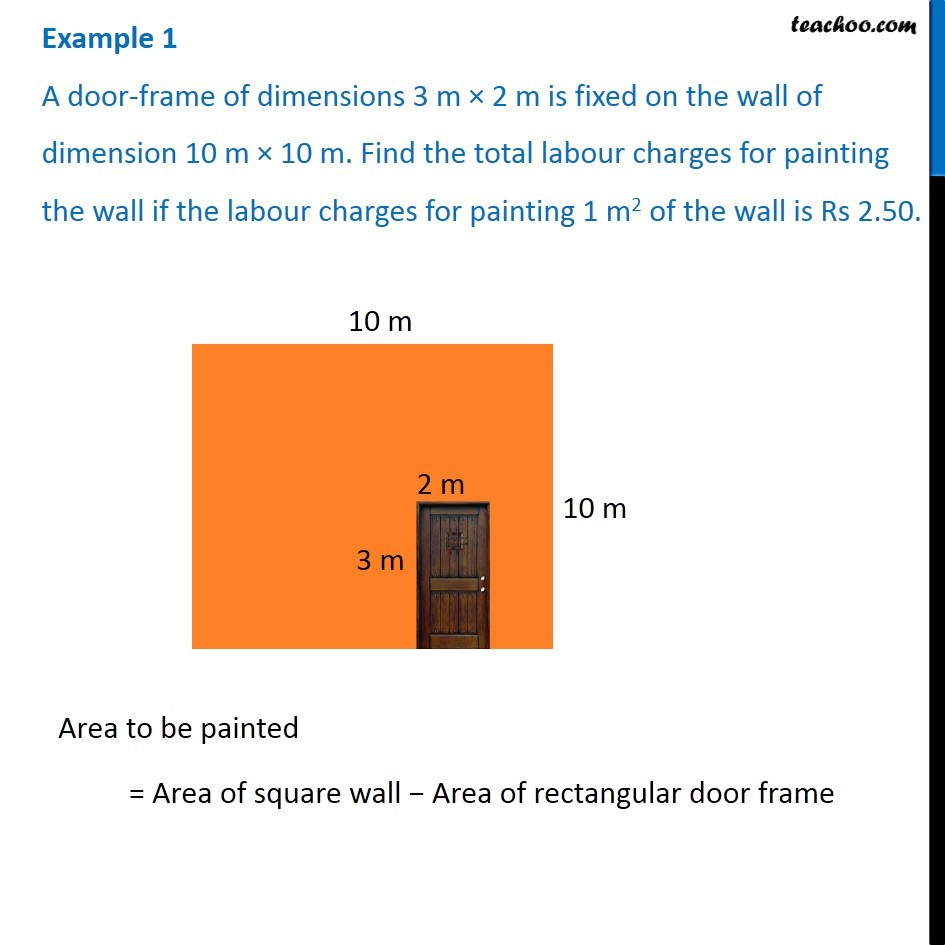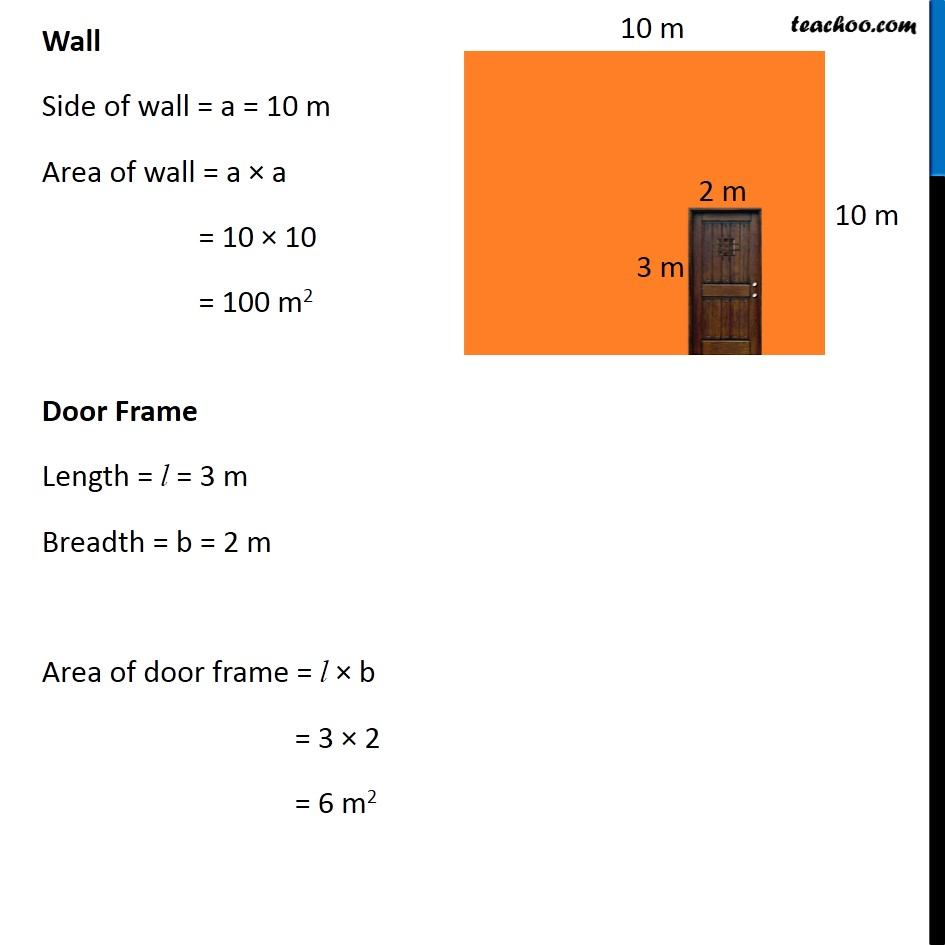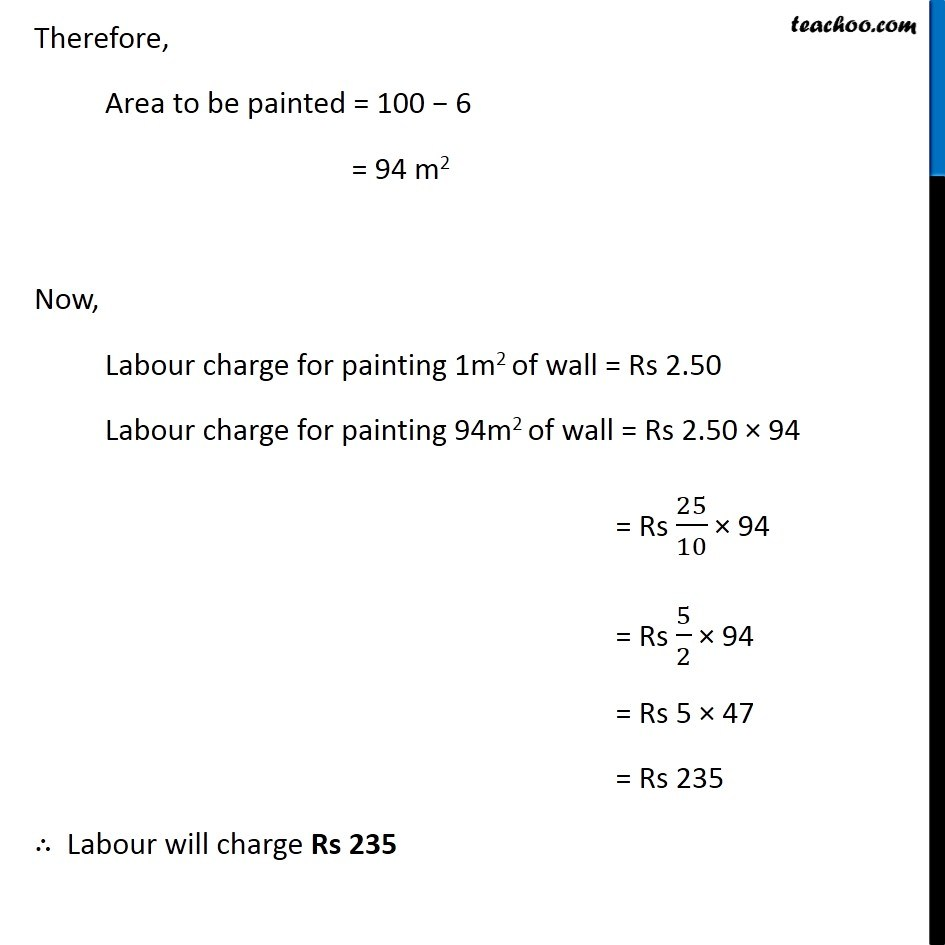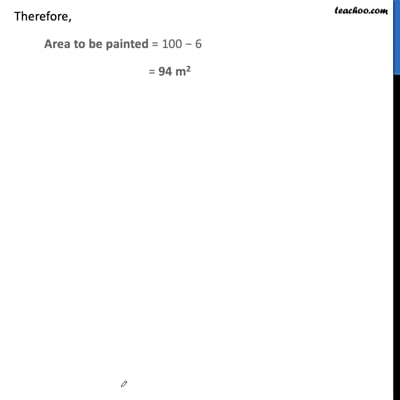Examples

Chapter 11 Class 7 Perimeter and Area
Serial order wiseThis video is only available for Teachoo black users

Get live Maths 1-on-1 Classs - Class 6 to 12

### Transcript

Example 1 A door-frame of dimensions 3 m × 2 m is fixed on the wall of dimension 10 m × 10 m. Find the total labour charges for painting the wall if the labour charges for painting 1 m2 of the wall is Rs 2.50. Area to be painted = Area of square wall − Area of rectangular door frame Wall Side of wall = a = 10 m Area of wall = a × a = 10 × 10 = 100 m2 Door Frame Length = l = 3 m Breadth = b = 2 m Area of door frame = l × b = 3 × 2 = 6 m2 Therefore, Area to be painted = 100 − 6 = 94 m2 Now, Labour charge for painting 1m2 of wall = Rs 2.50 Labour charge for painting 94m2 of wall = Rs 2.50 × 94 = Rs 25/10 × 94 = Rs 5/2 × 94 = Rs 5 × 47 = Rs 235 ∴ Labour will charge Rs 235